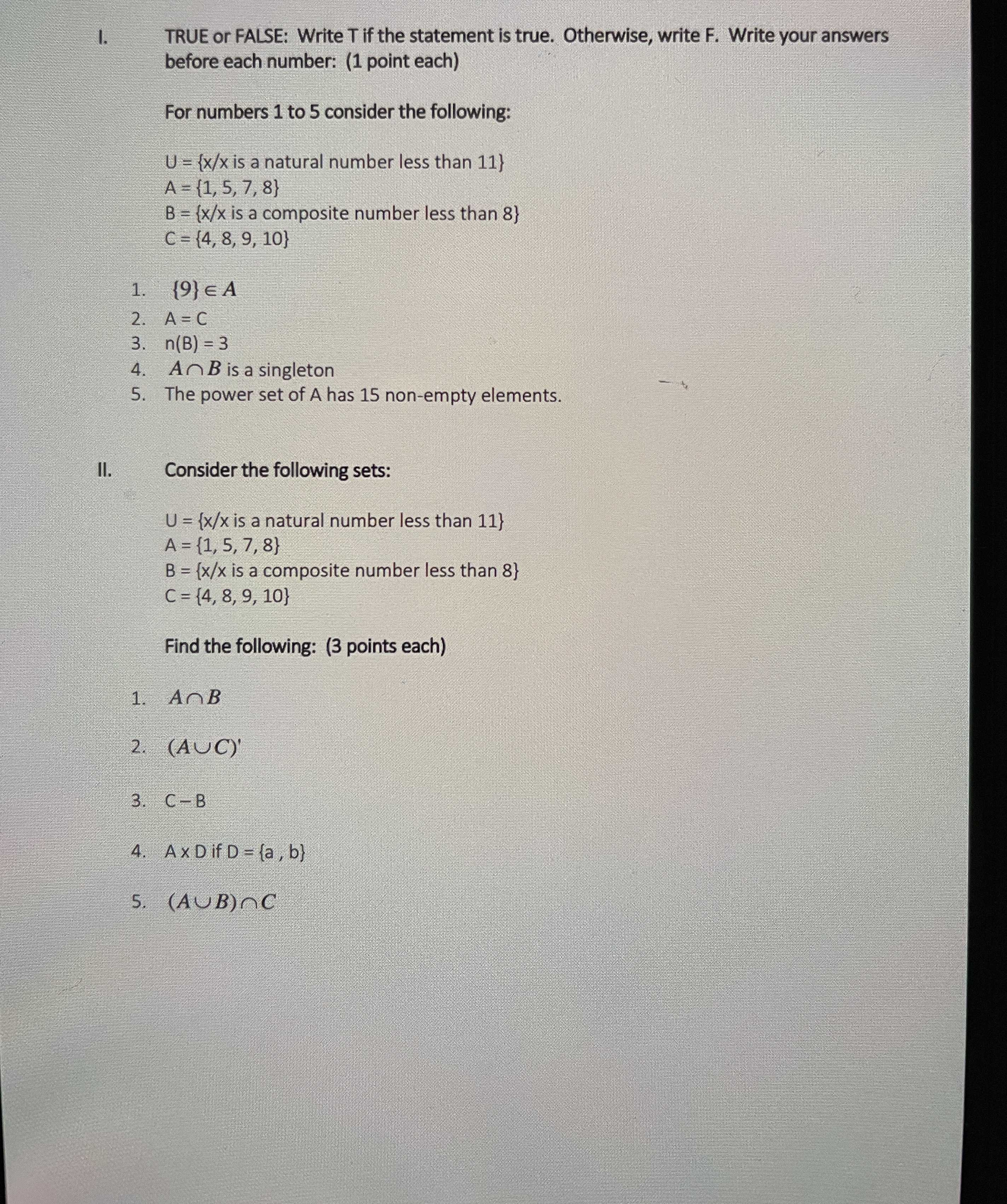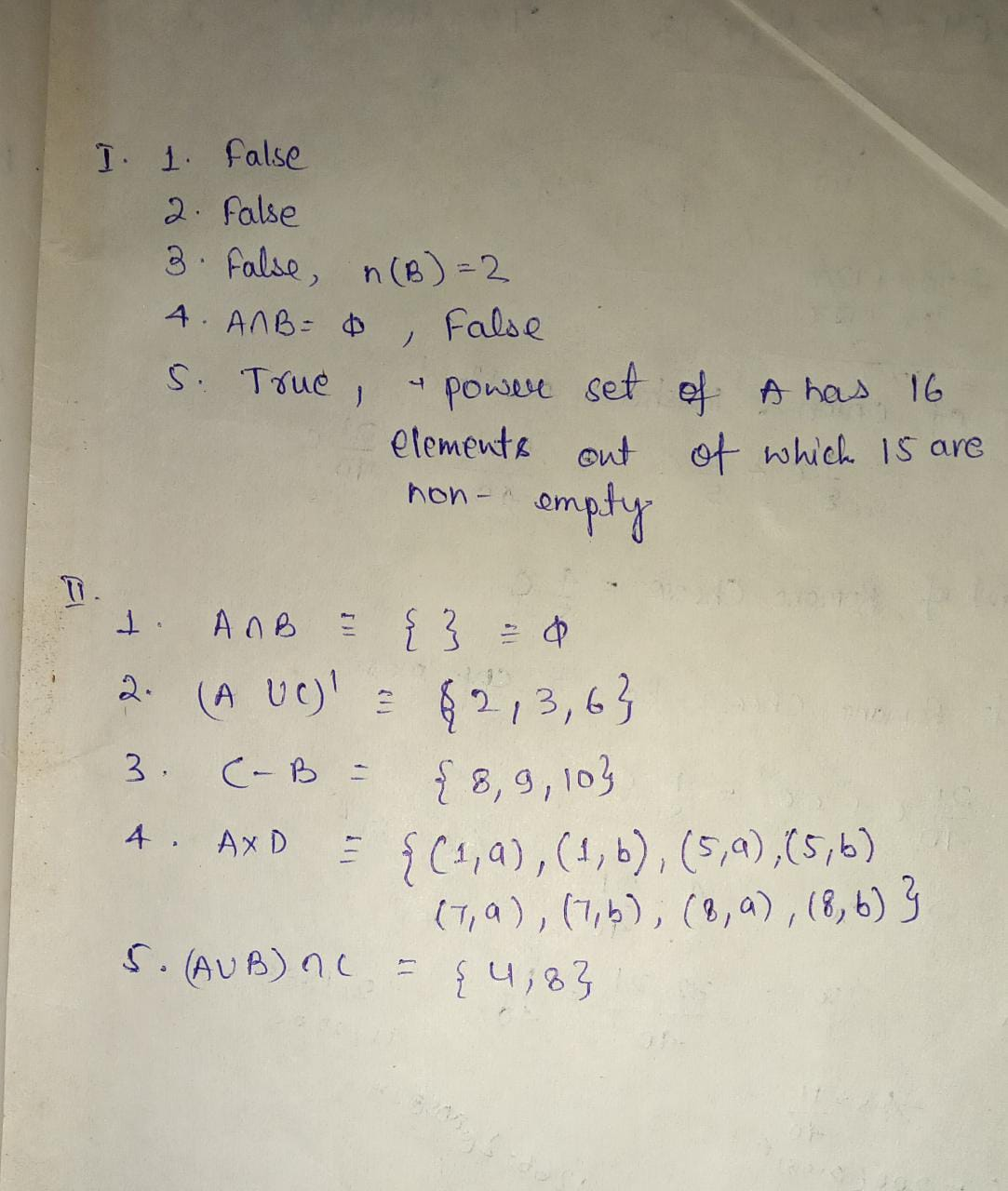### ¿Todavía tienes preguntas de matemáticas?

Pregunte a nuestros tutores expertos
Algebra
Pregunta1. TRUE or FALSE: Write T if the statement is true. Otherwise, write $$F$$ . Write your answers before each number:

$$U = \{ x / x$$ is a natural number less than $$11 \}$$ For numbers $$1$$ to $$5$$ consider the following:

$$A = \{ 1,5,7,8 \}$$

$$B = \{ x / x$$ is a composite number less than $$8 \}$$

$$C = \{ 4,8,9,10 \}$$

1. $$\{ 9 \} \in A$$

2. $$A = C$$ 3. $$n ( B ) = 3$$

4. $$A \cap B$$ is a singleton

5. The power set of $$A$$ has $$15$$ non-empty elements.

II. Consider the following sets:

$$U = \{ x / x$$ is a natural number less than $$11 \}$$

$$A = \{ 1,5,7,8 \}$$

$$B = \{ x / x$$ is a composite number less than $$8 \}$$

$$C = \{ 4,8,9,10 \}$$

Find the following:

1. $$A \cap B$$

2. $$( A \cup C ) ^ { \prime }$$

3. $$C - B$$ 4. $$A \times D$$ if $$D = \{ a , b \}$$

5. $$( A \cup B ) \cap C$$